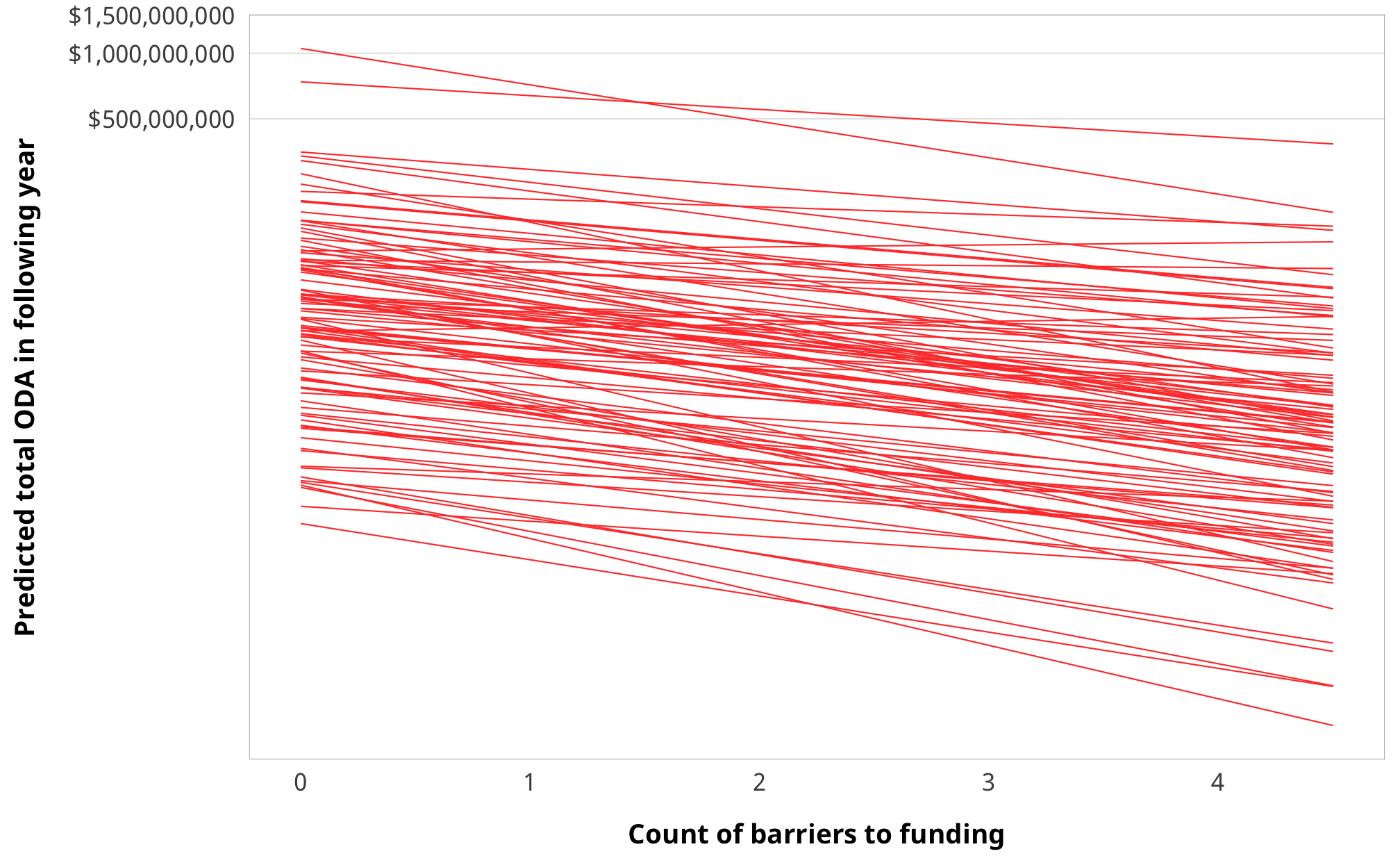library(tidyverse)
library(targets)
library(brms)
library(tidybayes)
library(bayesplot)
library(fixest)
library(broom)
library(broom.mixed)
library(modelsummary)
library(patchwork)
library(scales)
library(latex2exp)
library(kableExtra)
library(here)

color_scheme_set("viridisC")

my_seed <- 1234
set.seed(my_seed)

withr::with_dir(here::here(), {

# Data
df_country_aid_laws <- filter(df_country_aid, laws)

# Treatment models
m_oda_treatment_entry, m_oda_treatment_funding,
m_oda_treatment_ccsi))

# IPTW data
df_oda_iptw_entry, df_oda_iptw_funding,
df_oda_iptw_ccsi))

# Outcome models
m_oda_outcome_entry, m_oda_outcome_funding,
m_oda_outcome_ccsi))

# Results tables
})

## Weight models

• Numerator is treatment ~ lagged treatment + treatment history + non-varying confounders
• Denominator is treatment ~ lagged treatment + treatment history + lagged outcome + time-varying confounders + non-varying confounders

$sw = \prod^t_{t = 1} \frac{\phi(T_{it} | T_{i, t-1}, C_i)}{\phi(T_{it} | T_{i, t-1}, D_{i, t-1}, C_i)}$

### Priors for weight models

We use generic weakly informative priors for our model parameters:

• Intercept: $$\mathcal{N} (0, 10)$$
• Coefficients: $$\mathcal{N} (0, 2.5)$$
• Sigma: $$\operatorname{Cauchy} (0, 1)$$
pri_int <- ggplot() +
stat_function(fun = dnorm, args = list(mean = 0, sd = 10),
geom = "area", fill = "grey80", color = "black") +
labs(x = TeX("\\textbf{Intercept (β_0)}")) +
annotate(geom = "label", x = 0, y = 0.01, label = "N(0, 10)", size = pts(9)) +
xlim(-40, 40) +
theme_donors(prior = TRUE)

pri_coef <- ggplot() +
stat_function(fun = dnorm, args = list(mean = 0, sd = 2.5),
geom = "area", fill = "grey80", color = "black") +
labs(x = TeX("\\textbf{Coefficients (β_x)}")) +
annotate(geom = "label", x = 0, y = 0.04, label = "N(0, 3)", size = pts(9)) +
xlim(-10, 10) +
theme_donors(prior = TRUE)

pri_sigma <- ggplot() +
stat_function(fun = dcauchy, args = list(location = 0, scale = 1),
geom = "area", fill = "grey80", color = "black") +
labs(x = "σ") +
annotate(geom = "label", x = 5, y = 0.08, label = "Cauchy(0, 1)", size = pts(9)) +
xlim(0, 10) +
theme_donors(prior = TRUE)

(pri_int + pri_coef + pri_sigma)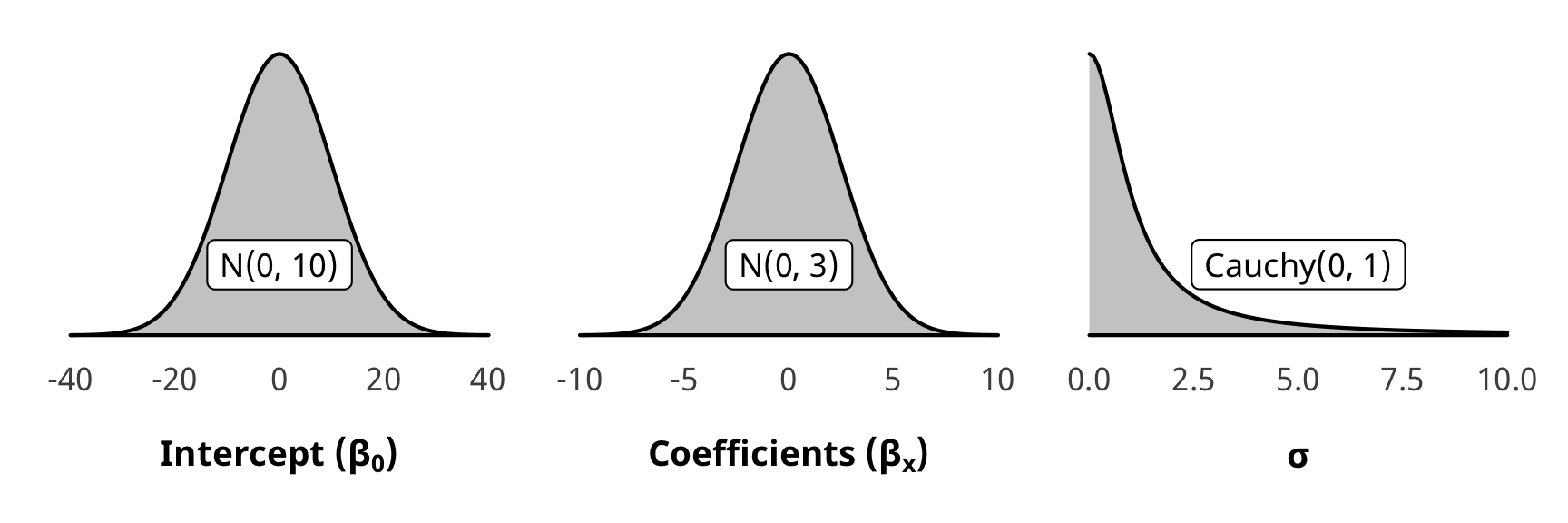### Check weight models

We check a couple of the numerator and denominator models for convergence and mixing and fit. They’re all pretty much the same

#### Numerator

pp_check(m_oda_treatment_total$model_num, type = "dens_overlay", nsamples = 10) + theme_donors()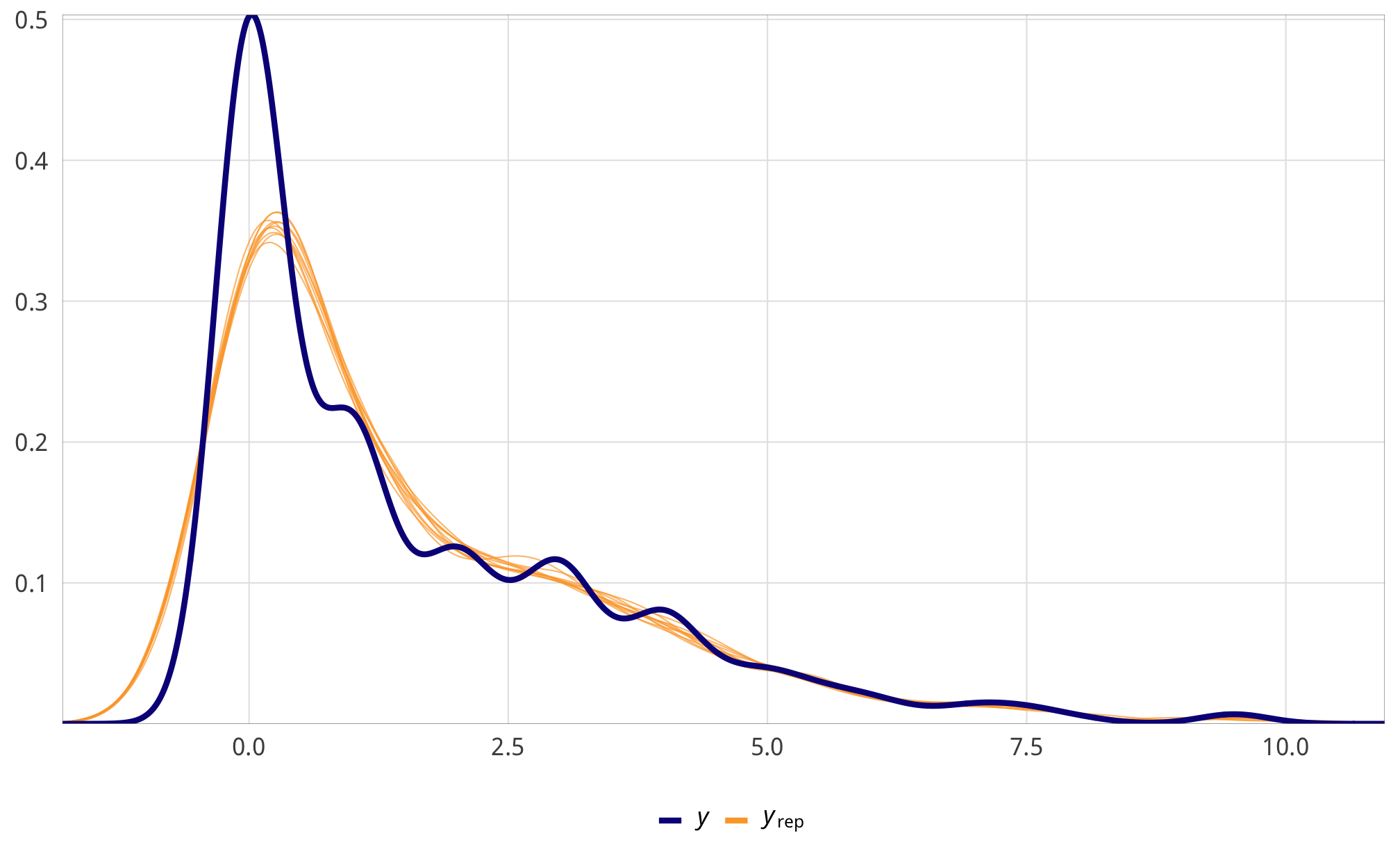m_oda_treatment_total$model_num %>%
select(-starts_with("r_gwcode"), -starts_with("z_"),
-starts_with("r_region"), -lp__, -iter) %>%
mcmc_trace() +
theme_donors() +
theme(legend.position = "right")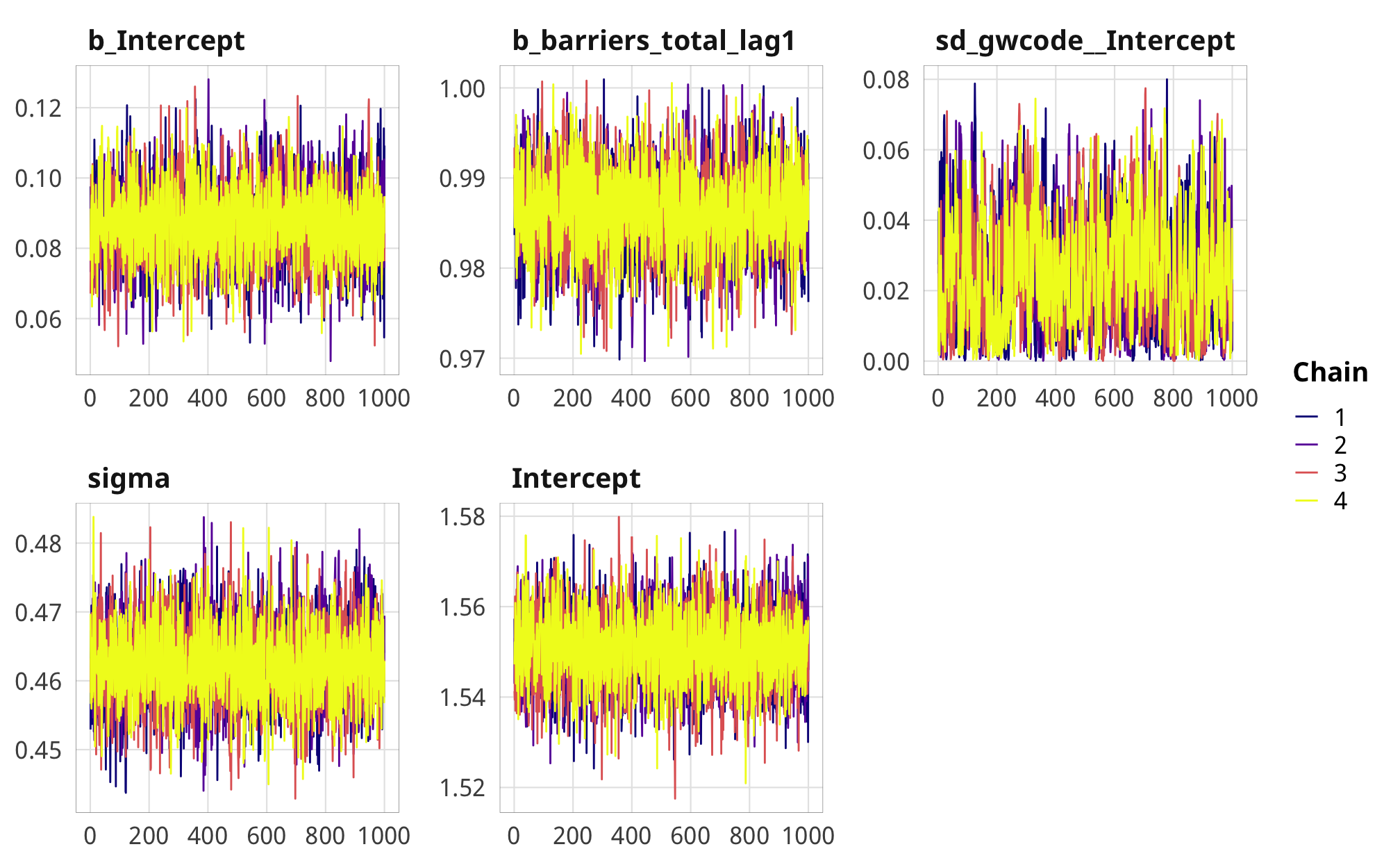#### Denominator

pp_check(m_oda_treatment_total$model_denom, type = "dens_overlay", nsamples = 10) + theme_donors()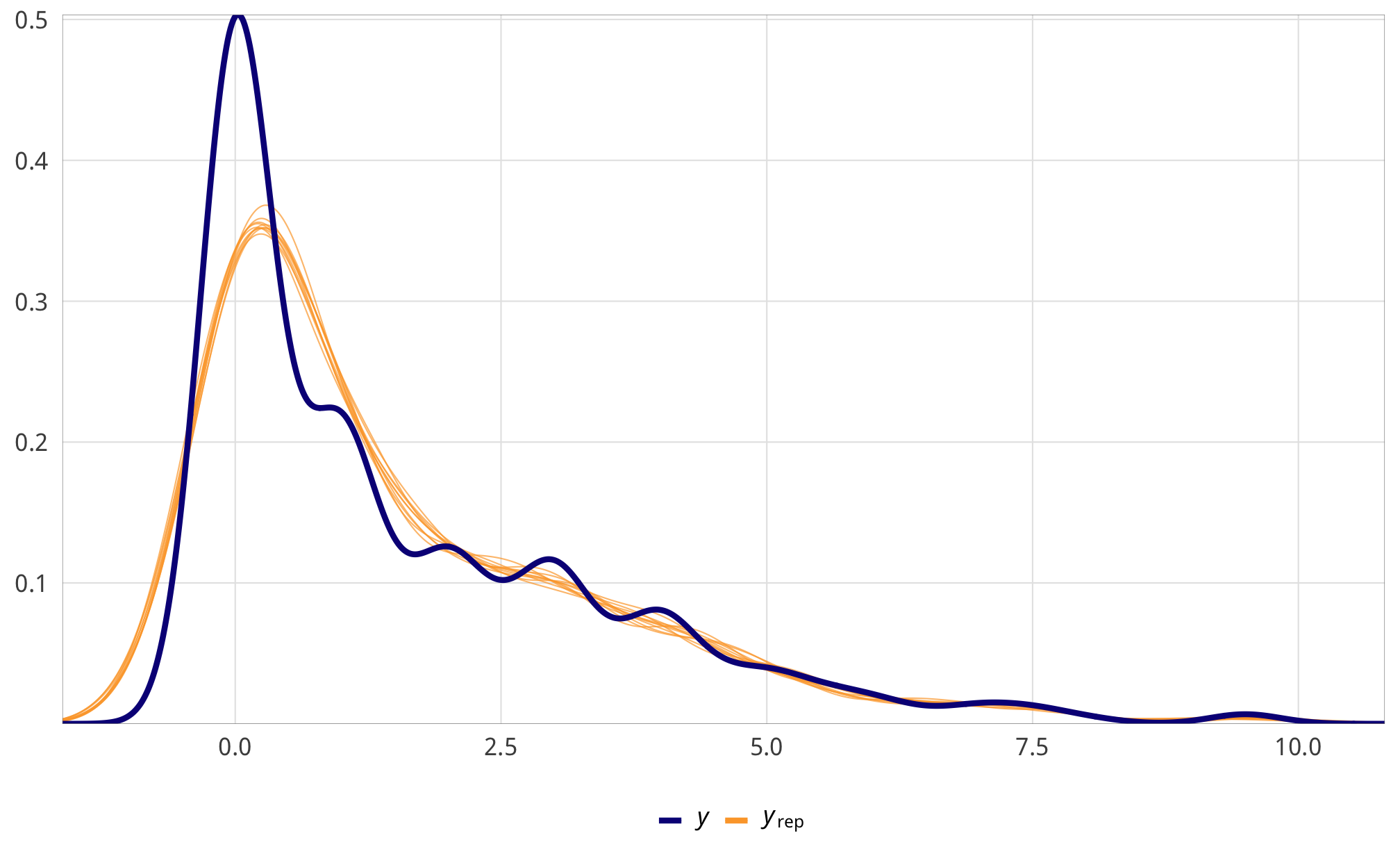m_oda_treatment_total$model_denom %>%
select(-starts_with("r_gwcode"), -starts_with("z_"),
-starts_with("r_region"), -lp__, -iter) %>%
mcmc_trace() +
theme_donors() +
theme(legend.position = "right")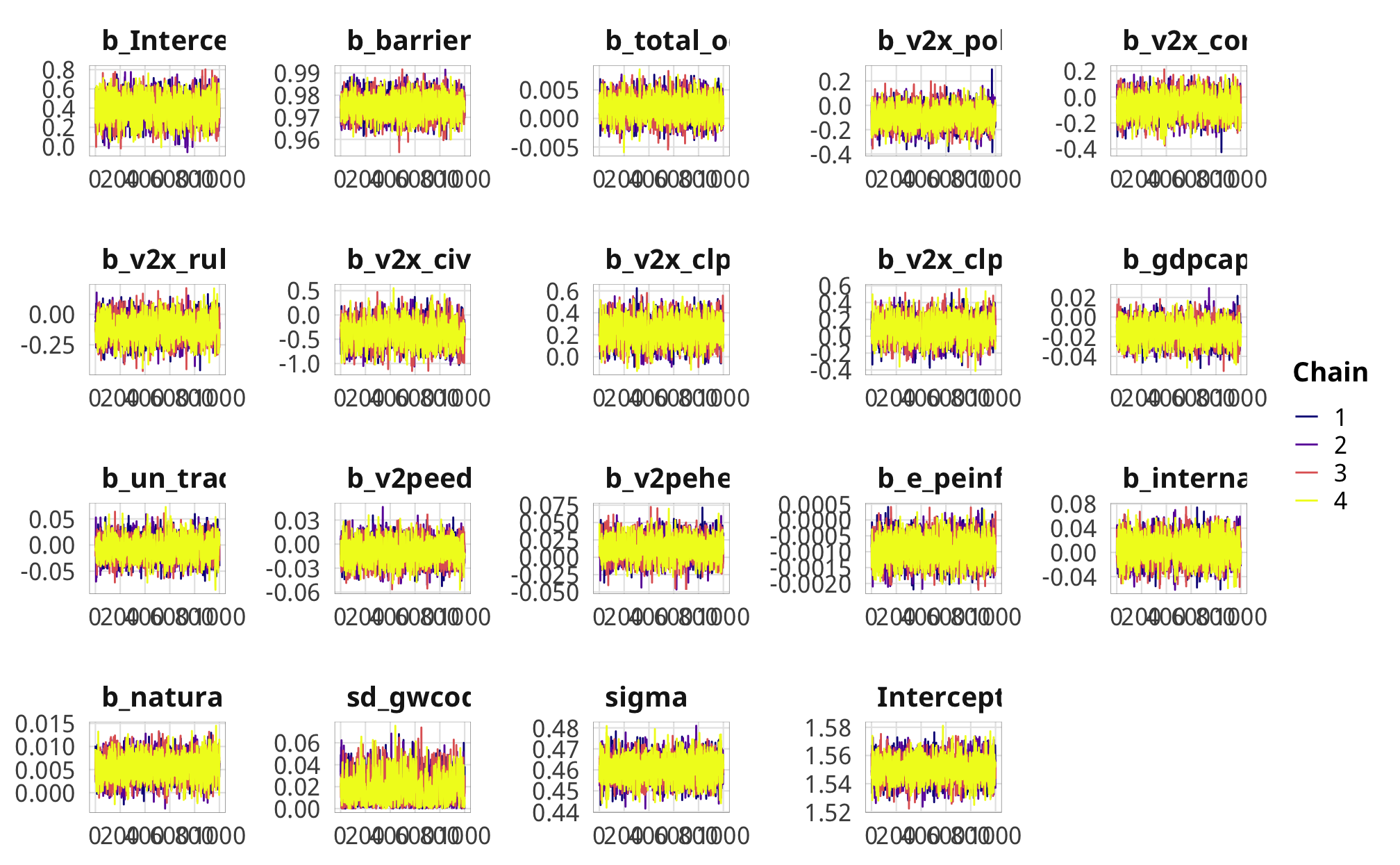### Check weights

IPTWs should generally have a mean of 1 and shouldn’t be too incredibly high. When they’re big, it’s a good idea to truncate them at some level. The truncation threshold matters substantially—as seen below, the ATE when using V-Dem’s core civil society index as the treatment varies substantially at different truncation thresholds.

Sweet.

summary(df_oda_iptw_total$iptw) ## Min. 1st Qu. Median Mean 3rd Qu. Max. ## 0.005716 0.854546 0.968154 1.060549 1.057472 10.769115 df_oda_iptw_total %>% ggplot(aes(x = iptw)) + geom_histogram(binwidth = 0.5, boundary = 0) + theme_donors()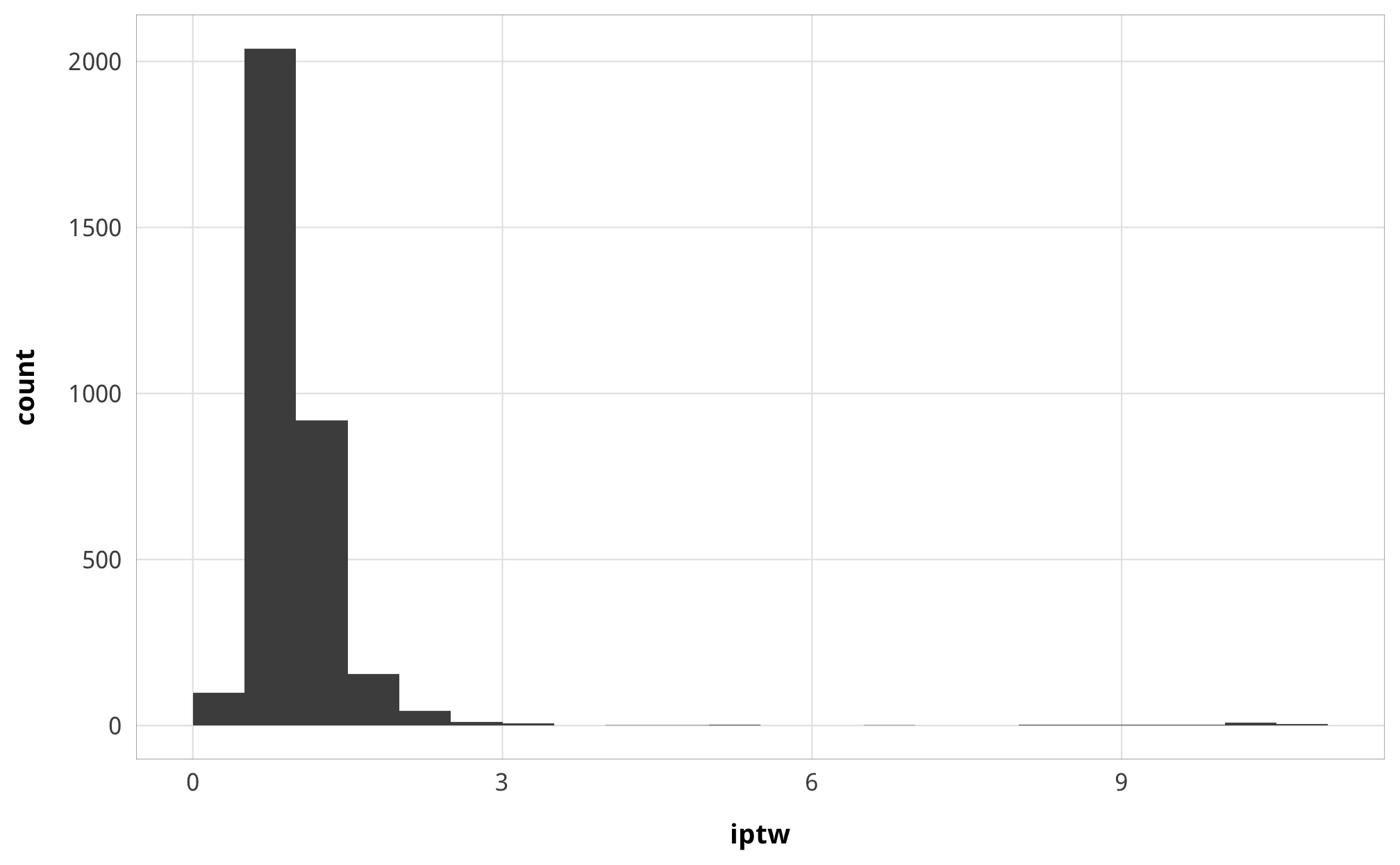#### Advocacy Lovely. summary(df_oda_iptw_advocacy$iptw)
##    Min. 1st Qu.  Median    Mean 3rd Qu.    Max.
##  0.1466  0.9180  0.9783  1.0159  1.0301  6.8357
df_oda_iptw_advocacy %>%
ggplot(aes(x = iptw)) +
geom_histogram(binwidth = 1, boundary = 0) +
theme_donors()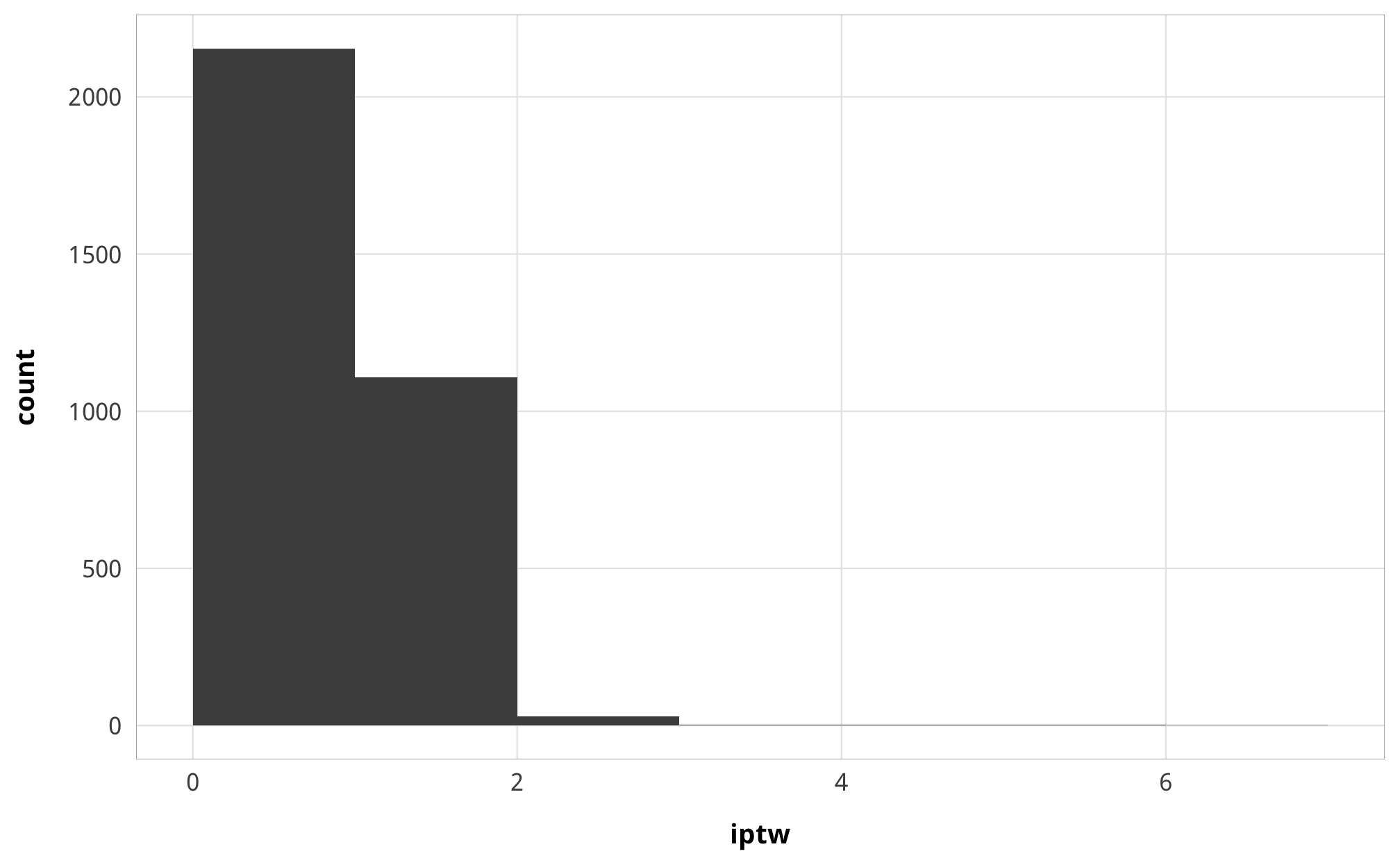Fantastic.

summary(df_oda_iptw_entry$iptw) ## Min. 1st Qu. Median Mean 3rd Qu. Max. ## 0.09036 0.91675 0.99041 1.01992 1.05431 4.22770 df_oda_iptw_entry %>% ggplot(aes(x = iptw)) + geom_histogram(binwidth = 0.5, boundary = 0) + theme_donors()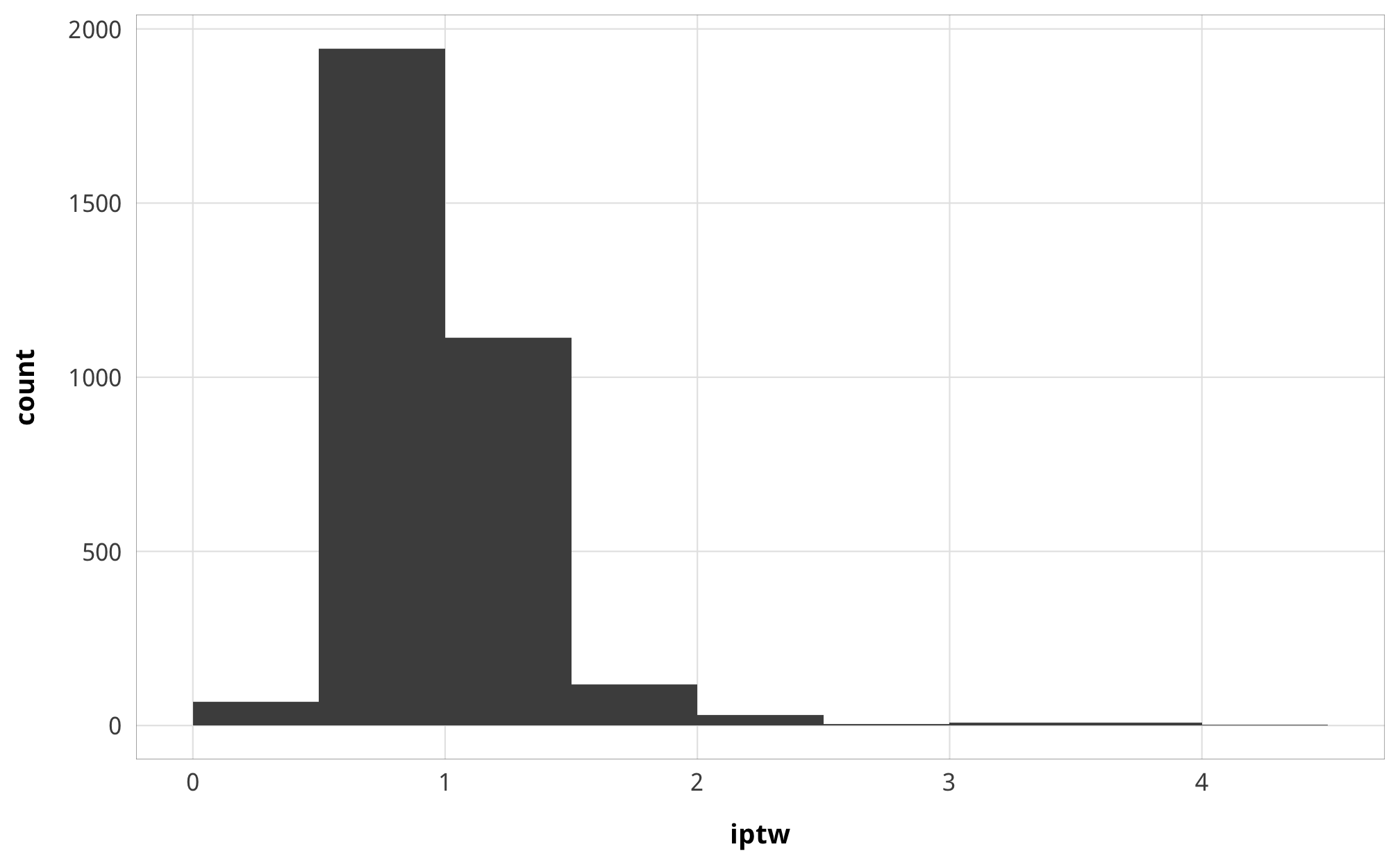#### Funding Slightly concerning maybe (though the median is great). summary(df_oda_iptw_funding$iptw)
##      Min.   1st Qu.    Median      Mean   3rd Qu.      Max.
##  0.003089  0.868638  0.958812  1.089450  1.011633 27.053270
df_oda_iptw_funding %>%
ggplot(aes(x = iptw)) +
geom_histogram(binwidth = 5, boundary = 0) +
theme_donors()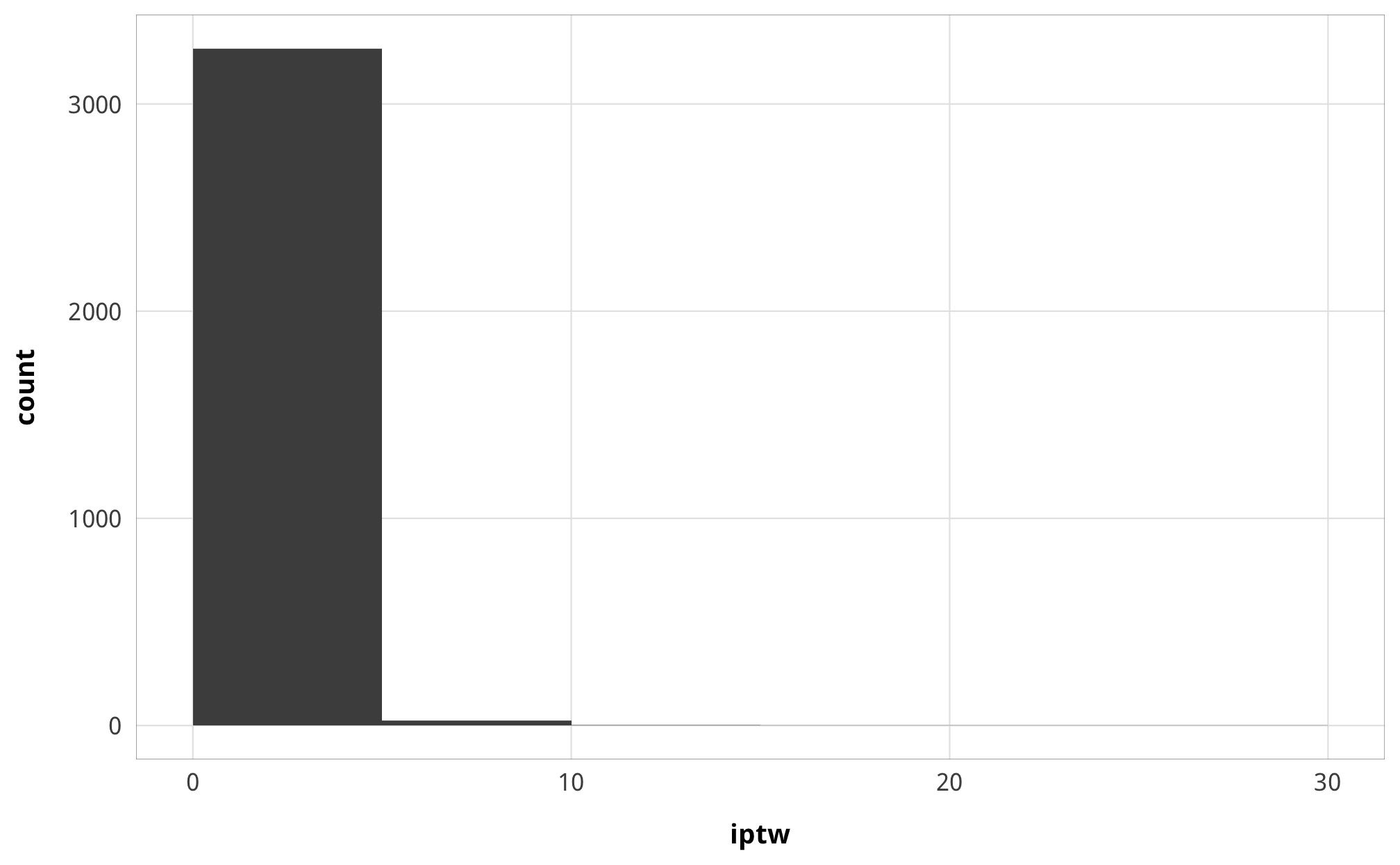#### Core civil society index

lol these blow up. Check out the maximum:

summary(df_oda_iptw_ccsi$iptw) ## Min. 1st Qu. Median Mean 3rd Qu. Max. ## 0.000e+00 0.000e+00 0.000e+00 9.932e+09 1.000e+00 3.531e+12 We truncate at four different thresholds: 100, 500, 1,000, and 5,000: plot_iptw_ccsi <- bind_rows( df_oda_iptw_ccsi %>% mutate(threshold = 100, iptw = ifelse(iptw > 100, 100, iptw)), df_oda_iptw_ccsi %>% mutate(threshold = 500, iptw = ifelse(iptw > 500, 500, iptw)), df_oda_iptw_ccsi %>% mutate(threshold = 1000, iptw = ifelse(iptw > 1000, 1000, iptw)), df_oda_iptw_ccsi %>% mutate(threshold = 5000, iptw = ifelse(iptw > 5000, 5000, iptw)) ) %>% mutate(threshold = paste0("Truncated at ", comma(threshold)), threshold = fct_inorder(threshold)) plot_iptw_ccsi %>% ggplot(aes(x = iptw)) + geom_histogram(bins = 50, boundary = 0) + facet_wrap(vars(threshold), scales = "free_x") + theme_donors()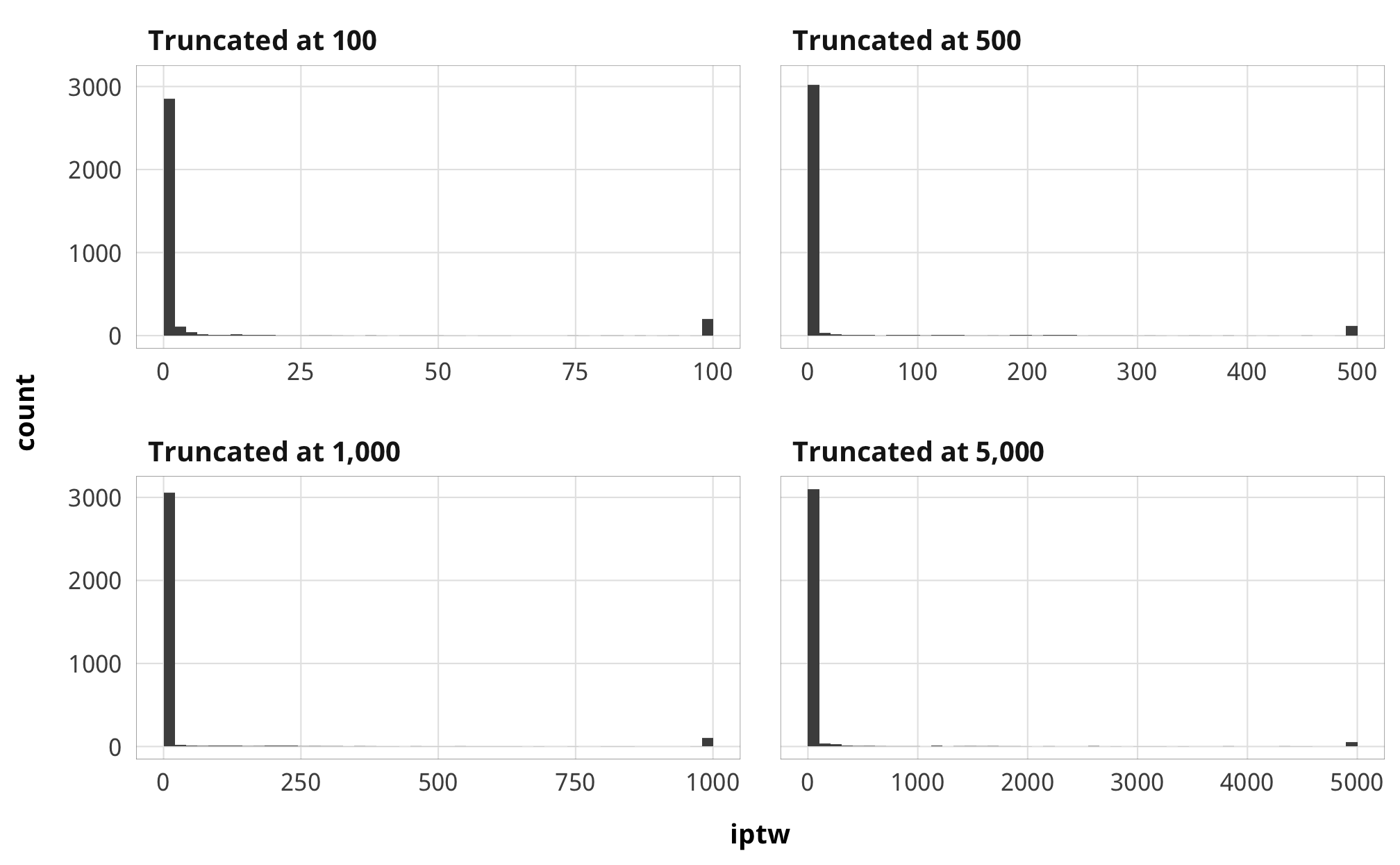### Results These are less important since we just use these treatment models to generate weights and because interpreting each coefficient when trying to isolate casual effects is unimportant and a “nuisance”. But here are the results anyway: #### Numerator models # model_names <- paste0("(", 1:length(models_tbl_h1_treatment_num), ")") model_names <- 1:length(models_tbl_h1_treatment_num) names(models_tbl_h1_treatment_num) <- model_names treatment_names <- c("Treatment", "Total barriers (t)", "Barriers to advocacy (t)", "Barriers to entry (t)", "Barriers to funding (t)", "CCSI (t)") treatment_rows <- as.data.frame(t(treatment_names)) attr(treatment_rows, "position") <- 1 coef_list_num <- list( "b_barriers_total_lag1" = "Treatment (t − 1)", "b_advocacy_lag1" = "Treatment (t − 1)", "b_entry_lag1" = "Treatment (t − 1)", "b_funding_lag1" = "Treatment (t − 1)", "b_v2xcs_ccsi_lag1" = "Treatment (t − 1)", "b_Intercept" = "Intercept" ) modelsummary(models_tbl_h1_treatment_num, statistic = "[{conf.low}, {conf.high}]", coef_map = coef_list_num, add_rows = treatment_rows, gof_omit = "ELPD", escape = FALSE, notes = list("Posterior means; 95% credible intervals in brackets")) 1 2 3 4 5 Treatment Total barriers (t) Barriers to advocacy (t) Barriers to entry (t) Barriers to funding (t) CCSI (t) Treatment (t − 1) 0.987 0.992 0.970 0.990 0.818 [0.977, 0.996] [0.983, 1.000] [0.957, 0.981] [0.980, 0.999] [0.799, 0.837] Intercept 0.087 0.015 0.051 0.030 0.119 [0.065, 0.108] [0.010, 0.020] [0.039, 0.064] [0.022, 0.039] [0.105, 0.134] Num.Obs. 3153 3153 3153 3153 3272 R2 0.941 0.949 0.929 0.940 0.958 R2 Marg. 0.941 0.949 0.928 0.940 0.904 LOOIC 4142.4 -4303.9 -13.2 -380.0 -9360.3 LOOIC s.e. 547.6 633.6 458.6 702.5 341.2 WAIC 4146.6 -4301.5 -12.8 -364.6 -9357.7 RMSE 1.33 0.34 0.66 0.60 0.33 Posterior means; 95% credible intervals in brackets #### Denominator models # model_names <- paste0("(", 1:length(models_tbl_h1_treatment_denom), ")") model_names <- 1:length(models_tbl_h1_treatment_denom) names(models_tbl_h1_treatment_denom) <- model_names treatment_names <- c("Treatment", "Total barriers (t)", "Barriers to advocacy (t)", "Barriers to entry (t)", "Barriers to funding (t)", "CCSI (t)") treatment_rows <- as.data.frame(t(treatment_names)) attr(treatment_rows, "position") <- 1 coef_list_denom <- list( "b_barriers_total_lag1" = "Treatment (t − 1)", "b_advocacy_lag1" = "Treatment (t − 1)", "b_entry_lag1" = "Treatment (t − 1)", "b_funding_lag1" = "Treatment (t − 1)", "b_v2xcs_ccsi_lag1" = "Treatment (t − 1)", "b_v2x_polyarchy" = "Polyarchy", "b_v2x_corr" = "Corruption index", "b_v2x_rule" = "Rule of law index", "b_v2x_civlib" = "Civil liberties index", "b_v2x_clphy" = "Physical violence index", "b_v2x_clpriv" = "Private civil liberties index", "b_gdpcap_log" = "Log GDP/capita", "b_un_trade_pct_gdp" = "Percent of GDP from trade", "b_v2peedueq" = "Educational equality index", "b_v2pehealth" = "Health equality index", "b_e_peinfmor" = "Infant mortality rate", "b_internal_conflict_past_5TRUE" = "Internal conflict in past 5 years", "b_natural_dis_count" = "Count of natural disasters", "b_Intercept" = "Intercept" ) modelsummary(models_tbl_h1_treatment_denom, statistic = "[{conf.low}, {conf.high}]", coef_map = coef_list_denom, add_rows = treatment_rows, gof_omit = "ELPD", escape = FALSE, notes = list("Posterior means; 95% credible intervals in brackets")) 1 2 3 4 5 Treatment Total barriers (t) Barriers to advocacy (t) Barriers to entry (t) Barriers to funding (t) CCSI (t) Treatment (t − 1) 0.975 0.987 0.965 0.977 0.489 [0.965, 0.985] [0.977, 0.995] [0.953, 0.975] [0.967, 0.988] [0.463, 0.514] Polyarchy -0.095 0.014 -0.079 -0.040 0.003 [-0.258, 0.070] [-0.032, 0.058] [-0.171, 0.011] [-0.124, 0.039] [-0.027, 0.031] Corruption index -0.073 -0.019 -0.028 -0.030 -0.022 [-0.246, 0.089] [-0.062, 0.028] [-0.118, 0.056] [-0.112, 0.058] [-0.056, 0.013] Rule of law index -0.119 -0.048 -0.033 -0.036 0.031 [-0.289, 0.092] [-0.100, 0.001] [-0.132, 0.067] [-0.129, 0.064] [-0.009, 0.071] Civil liberties index -0.367 -0.109 -0.162 -0.059 0.894 [-0.860, 0.119] [-0.235, 0.027] [-0.444, 0.092] [-0.325, 0.160] [0.818, 0.975] Physical violence index 0.231 0.062 0.111 0.048 -0.231 [0.004, 0.448] [0.004, 0.118] [-0.010, 0.227] [-0.059, 0.157] [-0.266, -0.193] Private civil liberties index 0.083 0.045 0.067 -0.039 -0.195 [-0.182, 0.344] [-0.025, 0.113] [-0.073, 0.209] [-0.169, 0.089] [-0.237, -0.152] Log GDP/capita -0.015 -0.003 -0.005 -0.008 -0.008 [-0.036, 0.006] [-0.008, 0.003] [-0.016, 0.006] [-0.018, 0.003] [-0.014, -0.003] Percent of GDP from trade -0.006 0.005 -0.008 -0.002 -0.006 [-0.045, 0.036] [-0.006, 0.015] [-0.031, 0.012] [-0.023, 0.018] [-0.014, 0.002] Educational equality index -0.009 -0.001 0.000 -0.008 -0.007 [-0.036, 0.019] [-0.008, 0.006] [-0.015, 0.014] [-0.022, 0.005] [-0.012, -0.001] Health equality index 0.013 0.000 -0.001 0.013 0.000 [-0.017, 0.045] [-0.008, 0.008] [-0.017, 0.015] [-0.002, 0.029] [-0.007, 0.006] Infant mortality rate -0.001 0.000 0.000 0.000 0.000 [-0.002, 0.000] [0.000, 0.000] [-0.001, 0.000] [-0.001, 0.000] [0.000, 0.000] Internal conflict in past 5 years 0.005 0.004 0.001 0.001 0.008 [-0.037, 0.046] [-0.007, 0.015] [-0.022, 0.022] [-0.019, 0.022] [0.002, 0.014] Count of natural disasters 0.006 0.001 0.002 0.002 0.000 [0.000, 0.011] [0.000, 0.003] [-0.001, 0.004] [0.000, 0.005] [-0.001, 0.001] Intercept 0.394 0.070 0.138 0.185 0.084 [0.142, 0.647] [0.005, 0.134] [-0.002, 0.266] [0.062, 0.312] [0.022, 0.143] Num.Obs. 3153 3153 3153 3153 3272 R2 0.942 0.949 0.929 0.941 0.970 R2 Marg. 0.942 0.949 0.928 0.941 0.946 LOOIC 4119.2 -4295.7 -16.3 -401.4 -10374.1 LOOIC s.e. 534.1 630.3 454.6 683.5 266.6 WAIC 4124.7 -4284.2 -17.2 -388.1 -10370.8 RMSE 1.33 0.34 0.66 0.60 0.33 Posterior means; 95% credible intervals in brackets ## Outcome models ### Modeling choices We use logged ODA, leaded by one year, as our main outcome variable throughout this analysis. We toyed with different parameterizations of ODA because of its exponential-ish distribution. For instance, instead of logging the outcome, some papers use an $$\operatorname{arcisnh}$$ transformation, but then interpretation gets tricky. It’s possible to use Poisson/negative binomial/Gamma models with dollar outcomes that are sometimes zero, but these kinds of ensemble models get unwieldy. A simpler version of the Poisson-Gamma combination is to use a hurdle model, of which there are four types currently in brms. One of these is hurdle_lognormal(), which log-transforms the outcome variable automatically. It’s possible to create custom families like hurdle_gaussian(), but this is super hard to do. I have an implementation partially completed here, but I can’t get it to actually create posterior predictions. Ugh. So we could fit a hurdled lognormal model, which uses a logit model to predict 0/not 0, then uses a lognormal distribution to model the rest of the data. However, this requires specifying two different models with different data-generating processes and different treatment effects, which becomes immensely unwieldy when dealing with marginal structural models and IPTWs. To simplify things, we initially tried using an intercept-only model (i.e. outcome ~ 1) for the 0/not 0 logit model and then outcome | weights(iptw) ~ treatment for the rest of the data and this worked, and the posterior predictive graphs looked phenomenal. BUT conceptually this was doing weird stuff. We were essentially ignoring all the 0s and not incorporating any of the IPTWs (or even the treatment variable!) into the models. Mathematically, this felt equivalent to just doing filter(treatment != 0). So in the end, we go back to the standard workhorse of just logging the outcome. ### Interpreting outcome models tidy(m_oda_outcome_funding) %>% mutate(estimate_exp = exp(estimate)) %>% filter(term == "funding") %>% select(term, estimate, estimate_exp) ## # A tibble: 1 x 3 ## term estimate estimate_exp ## <chr> <dbl> <dbl> ## 1 funding -0.274 0.760 Because our outcome is logged, the coefficients (and the ultimate ATE) represents a percent change in the outcome. The exponentiated coefficients represent percent changes for each unit increase in X. For instance, in the funding model, $$\beta_1$$ is -0.274, while $$e^{\beta_1}$$ is 0.76. Like exponentiated logistic regression coefficients, everything here is centered around 1, meaning that there’s a -24.0% decrease in aid for every legal barrier added. This can be also be seen as a multiplier effect. If aid is$50 million, it changes to 38,013,337 (50,000,000 × 0.76027). Interpreting this coefficient gets a little trickier because we used a multilevel model with population and group effects. This $$\beta_1$$ here is the average effect for a median cluster at each level of X. See this incredible resource for a ton more details about different ways of piecing together population- and group-level effects in a lognormal model. ### Priors for outcome models There are a ton of moving parts in these outcome models. Here’s the full specification for the models and all priors: \begin{aligned} \log (\text{Aid}_{i,t}) &\sim \text{IPTW}_{i, t-1} \times \mathcal{N} (\mu_{i, t}, \sigma_m) & \text{[likelihood]}\\ \mu_{i, t} &\sim \alpha_{\text{country}[i]} + \delta_{\text{year}[k]} + \beta\ \text{Law}_{i, t-1} & \text{[marginal structural model]}\\ \alpha_j &\sim \mathcal{N} (\bar{\alpha}, \sigma_{\alpha}) \text{, for } j \text{ in } 1 .. J & \text{[country-specific intercepts]} \\ \delta_j &\sim \mathcal{N} (0, \sigma_{\delta}) \text{, for } j \text{ in } 1 .. J & \text{[year-specific intercepts]} \\ \ \\ \bar{\alpha} &\sim \mathcal{N} (0, 20) & \text{[prior population intercept]} \\ \beta &\sim \mathcal{N} (0, 3) & \text{[prior population effects]} \\ \sigma_m, \sigma_{\alpha}, \sigma_{\delta} &\sim \operatorname{Cauchy}(0, 1) & \text{[prior sd for errors]} \end{aligned} ### Check outcome models The traceplots all look good. Here’s one: m_oda_outcome_total %>% posterior_samples(add_chain = TRUE) %>% select(-starts_with("r_gwcode"), -starts_with("z_"), -starts_with("r_year"), -lp__, -iter) %>% mcmc_trace() + theme_donors()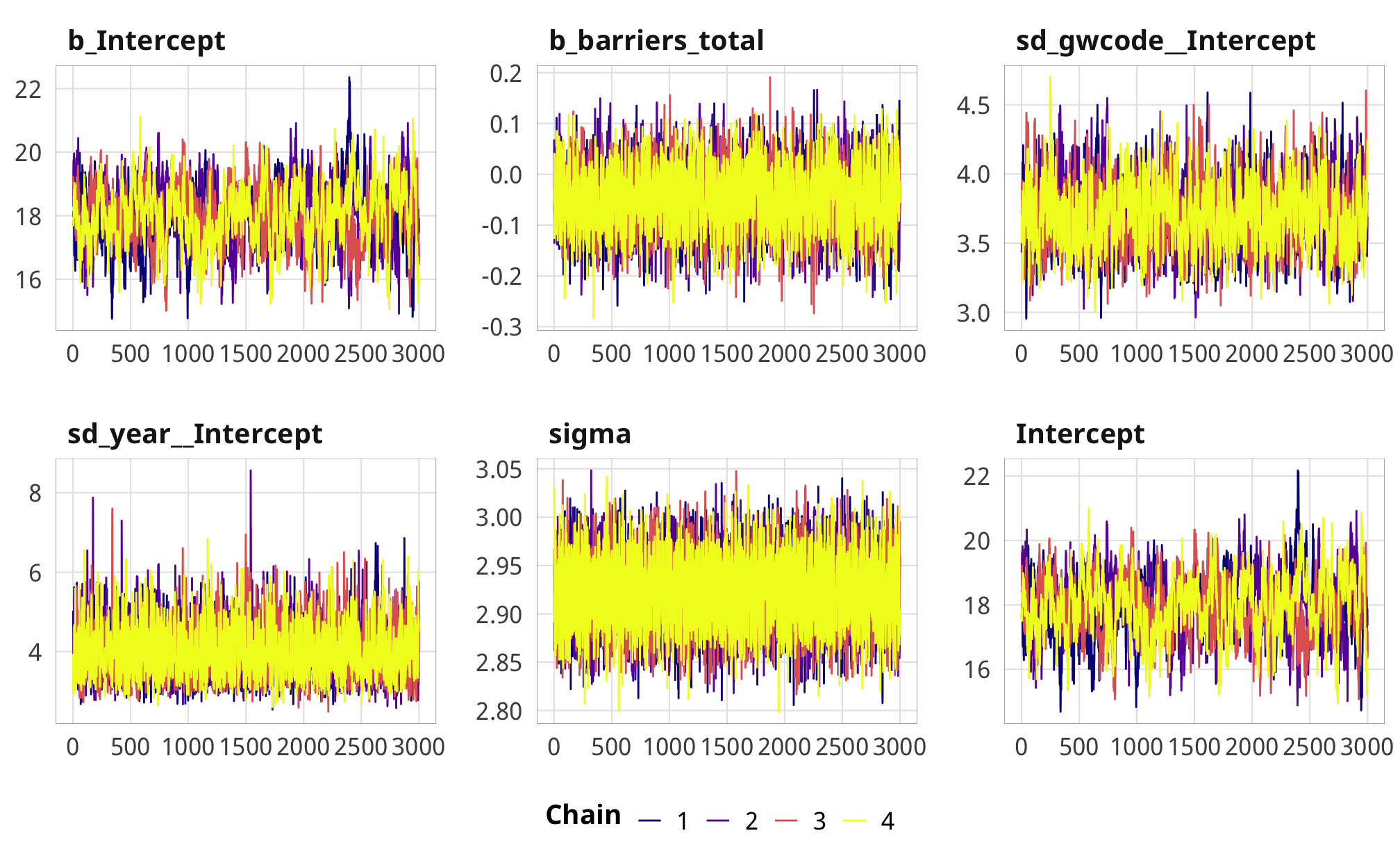### Results #### ATE plots coefs_nice <- tribble( ~.variable, ~var_nice, "b_barriers_total", "Additional law, t - 1", "b_advocacy", "Additional advocacy law, t - 1", "b_entry", "Additional entry law, t - 1", "b_funding", "Additional funding law, t - 1", "b_v2xcs_ccsi", "Core civil society index, t - 1" ) %>% mutate(var_nice = fct_inorder(var_nice)) plot_total_data <- gather_draws(m_oda_outcome_total, b_barriers_total) plot_advocacy_data <- gather_draws(m_oda_outcome_advocacy, b_advocacy) plot_entry_data <- gather_draws(m_oda_outcome_entry, b_entry) plot_funding_data <- gather_draws(m_oda_outcome_funding, b_funding) plot_total_aid_coefs <- bind_rows(plot_total_data, plot_advocacy_data, plot_entry_data, plot_funding_data) %>% left_join(coefs_nice, by = ".variable") rope_sd <- sd(df_oda_iptw_totaltotal_oda_log_lead1)

plot_rope <- tibble(xmin = -0.05 * rope_sd,
xmax = 0.05 * rope_sd)

ggplot(plot_total_aid_coefs, aes(x = .value, y = fct_rev(var_nice), fill = var_nice)) +
geom_vline(xintercept = 0) +
geom_rect(data = plot_rope, aes(xmin = xmin, xmax = xmax, ymin = -Inf, ymax = Inf),
fill = "#FFDC00", alpha = 0.3, inherit.aes = FALSE) +
annotate(geom = "label", x = 0, y = 0.65,
label = "Region of practical equivalence\n(± 0.05 × SD log foreign aid)",
size = pts(8)) +
stat_halfeye(.width = c(0.8, 0.95), alpha = 0.8, point_interval = "median_hdi") +
guides(fill = FALSE) +
scale_y_discrete() +
scale_fill_manual(values = c("#FF851B", "#85144b", "#3D9970", "#001f3f")) +
labs(x = "Change in log foreign aid in following year", y = NULL,
caption = "Point shows posterior median; lines show 80% and 95%\ncredible highest-density-interval") +
theme_donors()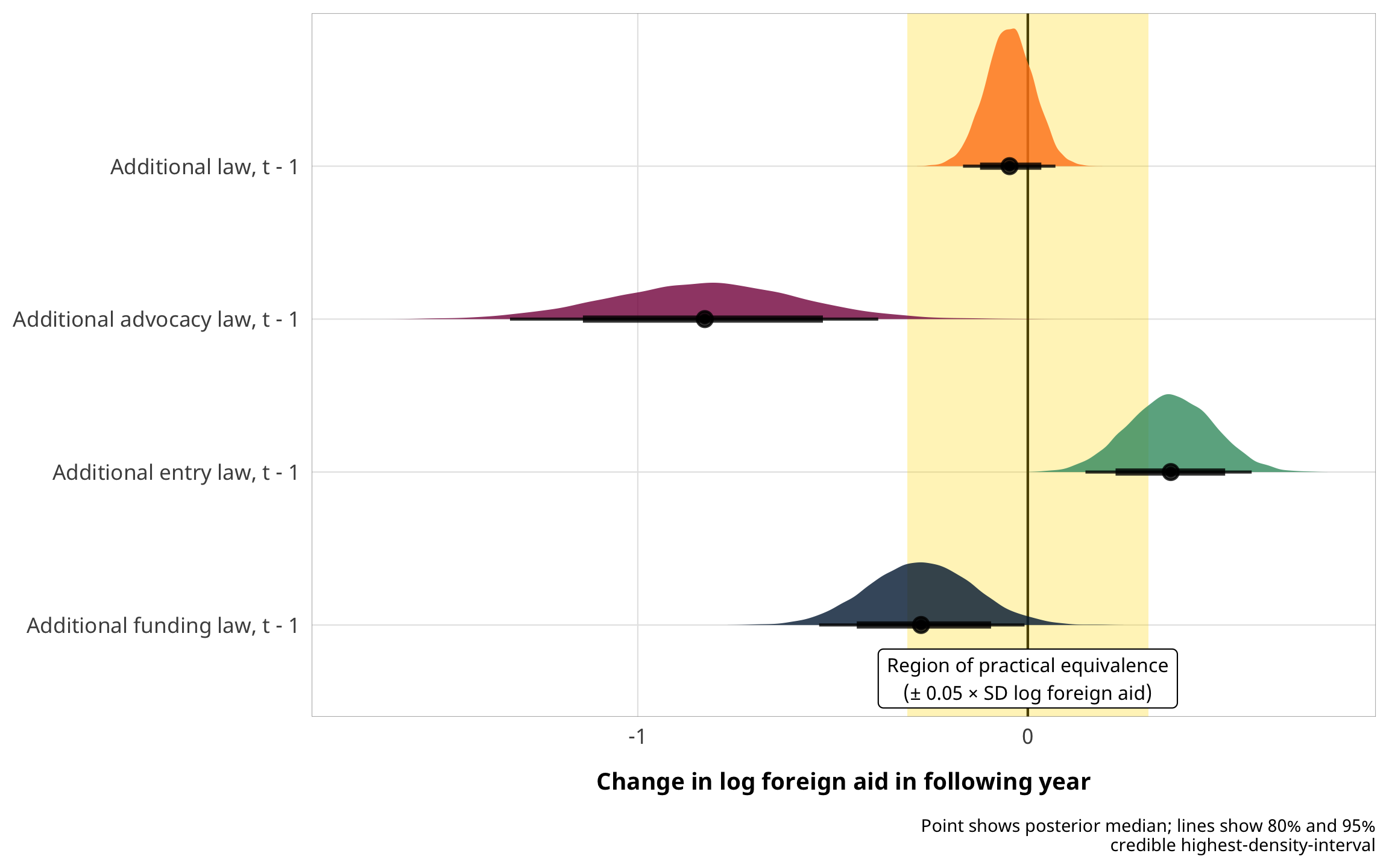plot_ccsi_coefs <- m_oda_outcome_ccsi %>%
map_dfr(~gather_draws(., b_v2xcs_ccsi), .id = "model") %>%
mutate(.value = .value / 10) %>%
mutate(model = comma(as.numeric(str_remove(model, "model_")))) %>%
mutate(model = fct_inorder(model)) %>%
left_join(coefs_nice, by = ".variable")

ggplot(plot_ccsi_coefs, aes(x = .value, y = fct_rev(model), fill = model)) +
stat_halfeye(.width = c(0.8, 0.95), point_interval = "median_hdi",
position = position_dodge(width = 0.5)) +
guides(fill = FALSE) +
labs(x = "Coefficient", y = "IPTW truncation point") +
theme_donors()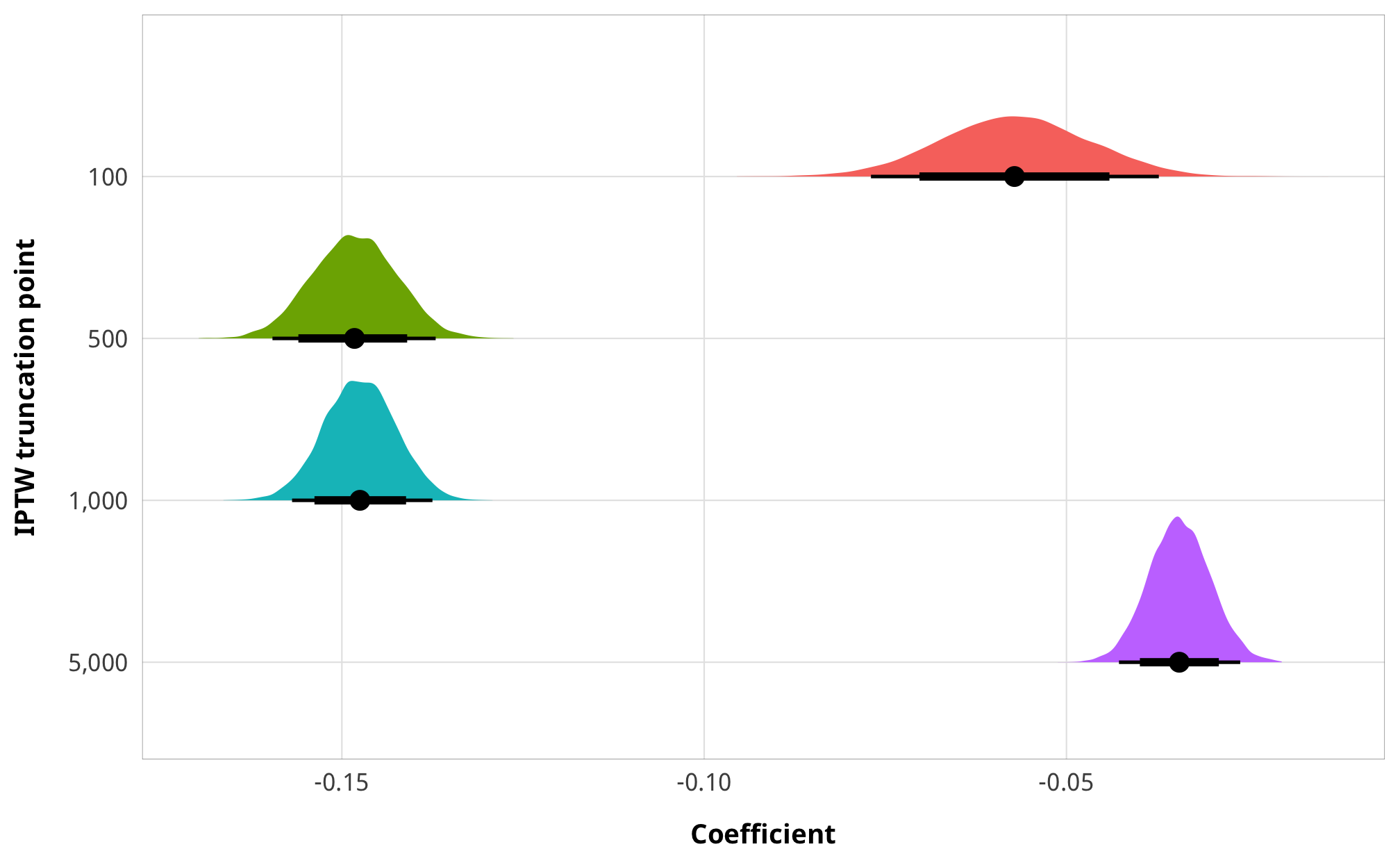#### Tables

# model_names <- paste0("(", 1:length(models_tbl_h1_outcome_dejure), ")")
model_names <- 1:length(models_tbl_h1_outcome_dejure)
names(models_tbl_h1_outcome_dejure) <- model_names

treatment_names <- c("Treatment", "Total barriers (t)", "Barriers to advocacy (t)",
"Barriers to entry (t)", "Barriers to funding (t)")
treatment_rows <- as.data.frame(t(treatment_names))
attr(treatment_rows, "position") <- 1

coef_list_outcome <- list(
"b_barriers_total" = "Treatment (t)",
"b_entry" = "Treatment (t)",
"b_funding" = "Treatment (t)",
"b_v2xcs_ccsi" = "Core civil society index (t)",
"b_Intercept" = "Intercept",
"sd_gwcode__Intercept" = "σ country intercepts",
"sd_year__Intercept" = "σ year intercepts"
)

modelsummary(models_tbl_h1_outcome_dejure,
statistic = "[{conf.low}, {conf.high}]",
coef_map = coef_list_outcome,
gof_omit = "ELPD",
escape = FALSE,
notes = list("Posterior means; 95% credible intervals in brackets")) %>%
add_header_above(c(" " = 1, "Outcome: ODA (t + 1)" = 4))
Outcome: ODA (t + 1)
1 2 3 4
Treatment Total barriers (t) Barriers to advocacy (t) Barriers to entry (t) Barriers to funding (t)
Treatment (t) -0.047 -0.828 0.367 -0.274
[-0.166, 0.071] [-1.328, -0.383] [0.148, 0.574] [-0.535, -0.008]
Intercept 18.035 18.180 17.632 17.983
[16.166, 19.811] [16.422, 19.856] [15.764, 19.328] [16.286, 19.662]
σ country intercepts 3.689 3.743 3.671 3.658
[3.258, 4.145] [3.312, 4.213] [3.251, 4.134] [3.238, 4.112]
σ year intercepts 3.931 3.881 3.959 3.893
[2.951, 5.235] [2.885, 5.147] [2.962, 5.288] [2.866, 5.192]
Num.Obs. 3293 3293 3293 3293
R2 0.740 0.743 0.738 0.738
R2 Marg. 0.000 0.005 0.003 0.002
LOOIC 17588.4 17380.8 17023.7 18112.3
LOOIC s.e. 292.6 235.9 229.1 336.5
WAIC 17587.5 17379.5 17022.9 18111.4
Posterior means; 95% credible intervals in brackets
truncation_thresholds <- names(models_tbl_h1_outcome_defacto) %>%
str_extract("_\\d+") %>%
str_remove("_") %>%
as.numeric() %>%
comma()

truncation_names <- c("IPTW truncation threshold", truncation_thresholds)
truncation_rows <- as.data.frame(t(truncation_names))
attr(truncation_rows, "position") <- 1

# model_names <- paste0("(", 1:length(models_tbl_h1_outcome_defacto), ")")
model_names <- 1:length(models_tbl_h1_outcome_defacto)
names(models_tbl_h1_outcome_defacto) <- model_names

modelsummary(models_tbl_h1_outcome_defacto,
statistic = "[{conf.low}, {conf.high}]",
coef_map = coef_list_outcome,
gof_omit = "ELPD",
escape = FALSE,
notes = list("Posterior means; 95% credible intervals in brackets")) %>%
add_header_above(c(" " = 1, "Outcome: ODA (t + 1)" = 4))
Outcome: ODA (t + 1)
1 2 3 4
IPTW truncation threshold 100 500 1,000 5,000
Core civil society index (t) -0.572 -1.483 -1.475 -0.344
[-0.770, -0.373] [-1.596, -1.371] [-1.569, -1.375] [-0.427, -0.260]
Intercept 18.773 19.444 19.379 18.567
[16.813, 20.508] [17.527, 21.340] [17.402, 21.331] [16.549, 20.559]
σ country intercepts 3.633 3.691 3.686 3.645
[3.228, 4.103] [3.235, 4.160] [3.256, 4.132] [3.226, 4.106]
σ year intercepts 4.230 4.323 4.398 4.486
[3.132, 5.606] [3.196, 5.772] [3.239, 5.802] [3.299, 5.938]
Num.Obs. 3298 3298 3298 3298
R2 0.688 0.688 0.689 0.693
R2 Marg. 0.001 0.004 0.004 0.000
LOOIC 87427.2 294827.6 497278.6 1627189.8
LOOIC s.e. 13004.5 52636.6 88637.7 289710.0
WAIC 95294.4 343009.5 588537.4 1973938.8
Posterior means; 95% credible intervals in brackets

#### Marginal effects

# brms::conditional_effects() can create spaghetti plots (single lines per
# sample), but it stores them in an attribute slot named "spaghetti", which is
# tricky to extract data from. This pulls the data frame from the attribute
make_spaghetti <- function(model) {
raw_effects <- conditional_effects(model, spaghetti = TRUE, nsamples = 100)[]
spaghetti_data <- attr(raw_effects, "spaghetti")
return(spaghetti_data)
}

make_spaghetti(m_oda_outcome_total) %>%
mutate(estimate__ = exp(estimate__)) %>%
ggplot(aes(x = effect1__, y = estimate__, group = sample__)) +
geom_line(size = 0.25, color = "#FF4136") +
scale_x_continuous(breaks = 0:9) +
scale_y_continuous(labels = dollar, trans = pseudo_log_trans()) +
guides(color = FALSE) +
labs(x = "Count of total legal barriers",
y = "Predicted total ODA in following year") +
theme_donors() +
theme(panel.grid.major.x = element_blank())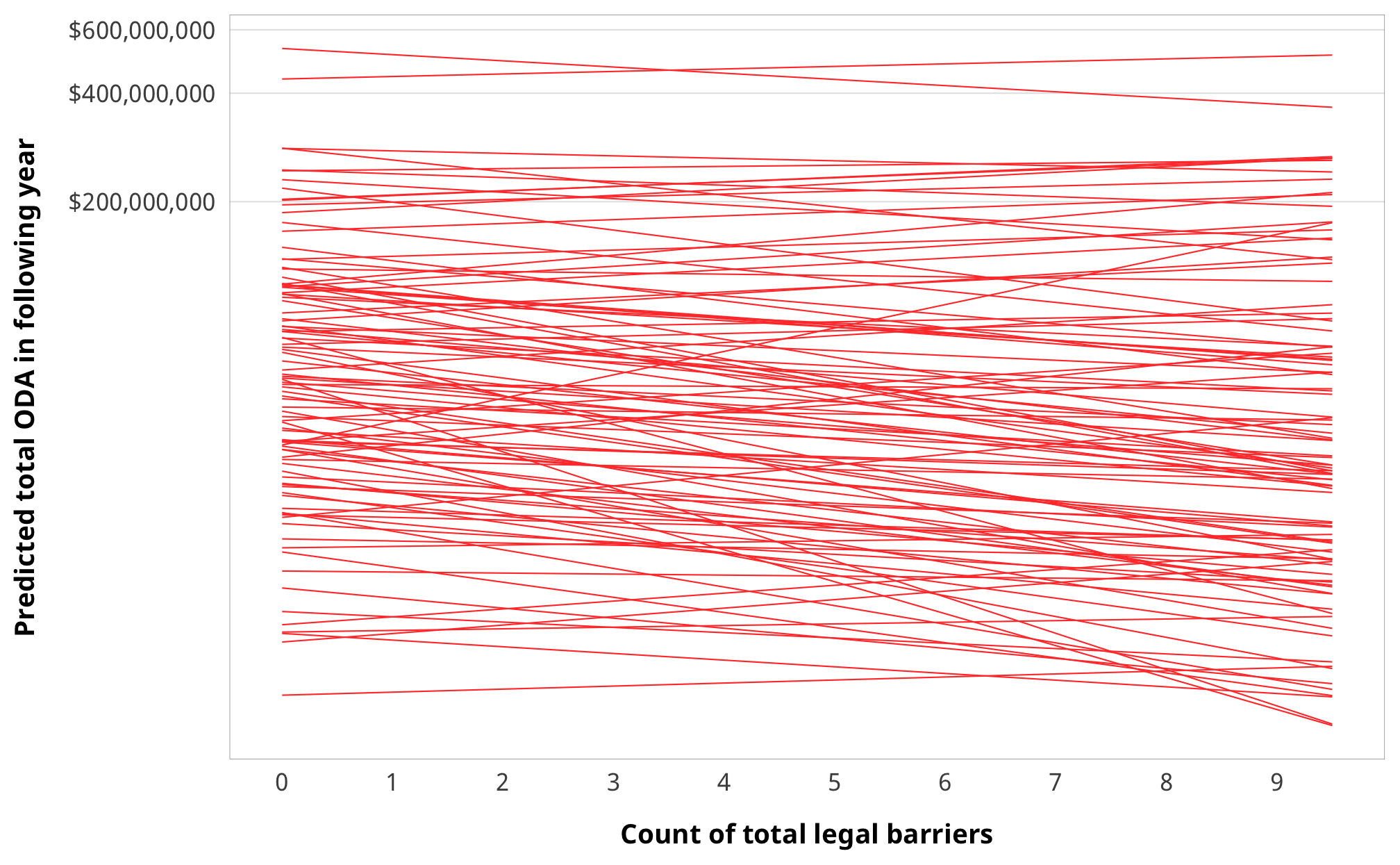make_spaghetti(m_oda_outcome_advocacy) %>%
mutate(estimate__ = exp(estimate__)) %>%
ggplot(aes(x = effect1__, y = estimate__, group = sample__)) +
geom_line(size = 0.25, color = "#FF4136") +
scale_x_continuous(breaks = 0:2) +
scale_y_continuous(labels = dollar, trans = pseudo_log_trans()) +
guides(color = FALSE) +
labs(x = "Count of barriers to advocacy",
y = "Predicted total ODA in following year") +
theme_donors() +
theme(panel.grid.major.x = element_blank())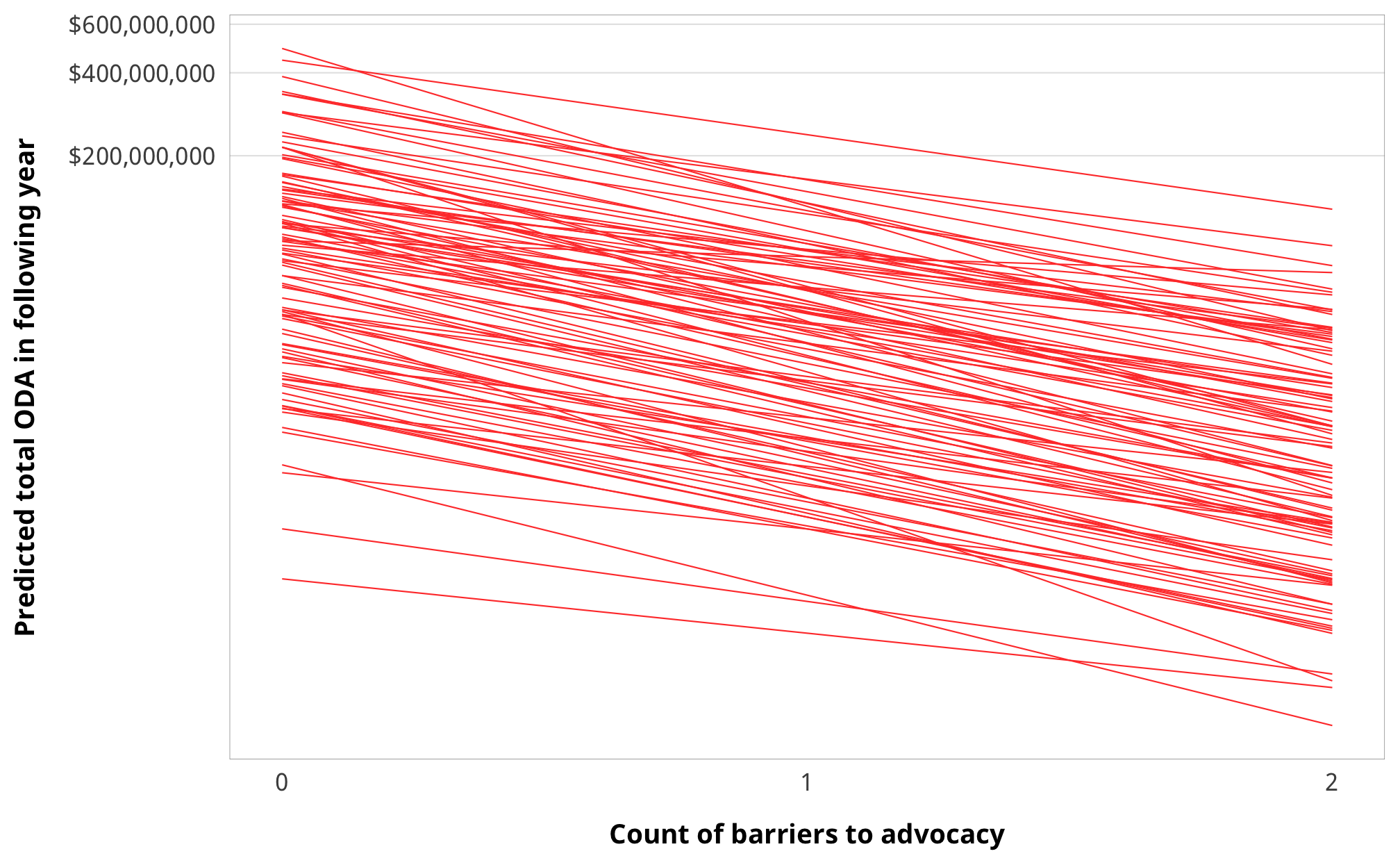make_spaghetti(m_oda_outcome_entry) %>%
mutate(estimate__ = exp(estimate__)) %>%
ggplot(aes(x = effect1__, y = estimate__, group = sample__)) +
geom_line(size = 0.25, color = "#FF4136") +
scale_x_continuous(breaks = 0:3) +
scale_y_continuous(labels = dollar, trans = pseudo_log_trans()) +
guides(color = FALSE) +
labs(x = "Count of barriers to entry",
y = "Predicted total ODA in following year") +
theme_donors() +
theme(panel.grid.major.x = element_blank())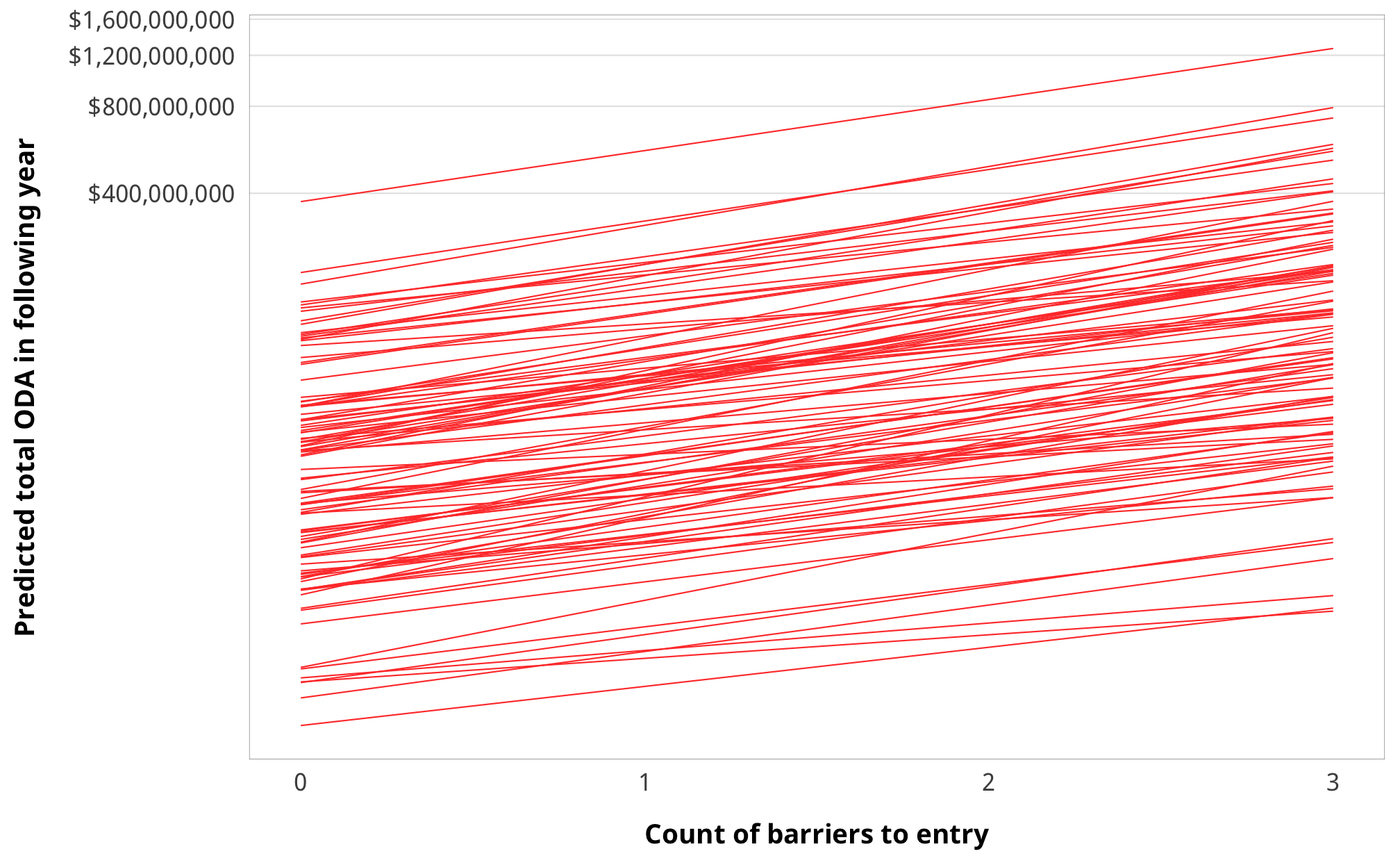make_spaghetti(m_oda_outcome_funding) %>%
mutate(estimate__ = exp(estimate__)) %>%
ggplot(aes(x = effect1__, y = estimate__, group = sample__)) +
geom_line(size = 0.25, color = "#FF4136") +
scale_x_continuous(breaks = 0:5) +
scale_y_continuous(labels = dollar, trans = pseudo_log_trans()) +
guides(color = FALSE) +
labs(x = "Count of barriers to funding",
y = "Predicted total ODA in following year") +
theme_donors() +
theme(panel.grid.major.x = element_blank())